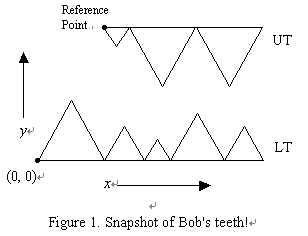JaWs

escription

"Sigh! Where are those good old bloody days?" Pondered Bob, the old shark, the former slayer of the deep blue waters, with his tears joining the infinite water of the ocean. Butchering for years, Bob's teeth has lost their regular shape, and the poor old shark is now in trouble closing his jaws. He wants to program his PDA to help him find the shape of his teeth when his jaws are closed and we want to help him write this program!

We name the sequence of Bob's lower teeth as LT and the sequence of his upper teeth as UT. For the sake of simplicity, consider LT as a sequence of adjacent equilateral triangles (i.e., with equal sides). All bases of the triangles lie on the same horizontal straight line. UT has a similar structure, except that the triangles are upside-down (Figure 1).Assume the left endpoint of the base of the leftmost tooth in LT has the coordinates (0, 0), so the bases of all triangles in LT lie on the x axis. We name the left end point of the base of the leftmost tooth in UT, the reference point. Initially, the coordinates of the reference point is given such that:

The tip of no two teeth in LT and UT have the same x coordinates,
UT is above LT, i.e. the y coordinate of the reference point is greater than zero,
LT and UT do not overlap at any point.

Given a placement of UT and LT conforming to the above conditions, UT starts falling downward such that the base of the triangles remain horizontal during its fall, i.e. UT does not rotate. UT continues to fall, until it touches some point in LT. At this time, UT slides downward (to the left or right) over LT until it cannot slide any further. During this motion, LT is fixed and UT never rotates. Note that UT may have an initial position such that it slides downward either from left or right, and falls below LT (Imagine the old shark in that state!). Also it is possible that the tips of some upper teeth finally pass the line y = 0 (the Dracula-style!). Your program should determine whether UT falls down from left or right, or otherwise, finds the final position of the reference point after it stops moving.
Input

The first line of the input file contains a single integer t (1 <= t <= 10), the number of test cases, followed by the input data for each test case. The first line of each test case contains integer L (1 <= L <= 10) the number of triangles in LT, followed by L lines each describing a triangle in LT, containing one integer number b (1 <= b <= 100), the side of the triangle. The next line of the input consists of three numbers x, y, and U. The first two numbers are the initial (x, y) coordinates of the reference point and are arbitrary real numbers. U is the number of triangles in UT (1 <= U <= 10). After this line, there are U lines each describing a triangle in LT, containing one integer number b (1 <= b <= 100), the side of the triangle.

In order to avoid floating-point arithmetic errors, you may assume that the input has the property that during the motion of UT, the distance between tips of any two triangles in LT and UT is never less than 0.1.
Output

There should be one line per test case containing a pair of real numbers, rounded to three digits after decimal point, which are x and y coordinates of the reference point after UT stops moving. If UT falls down from left of LT, the output line should contain the word WM, and if it falls down from the right of LT, it should be MW. The output is considered to be case-sensitive.
Sample Input

2
2
10
10
2 20 2
10
10
1
10
50 50 1
10

Sample Output

5.000 8.660
MW

1个回答